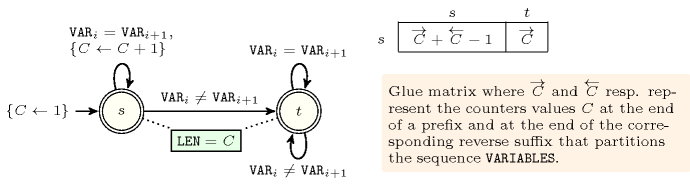## 5.216. length_last_sequence

Origin
Constraint

$\mathrm{𝚕𝚎𝚗𝚐𝚝𝚑}_\mathrm{𝚕𝚊𝚜𝚝}_\mathrm{𝚜𝚎𝚚𝚞𝚎𝚗𝚌𝚎}\left(\mathrm{𝙻𝙴𝙽},\mathrm{𝚅𝙰𝚁𝙸𝙰𝙱𝙻𝙴𝚂}\right)$

Arguments
 $\mathrm{𝙻𝙴𝙽}$ $\mathrm{𝚍𝚟𝚊𝚛}$ $\mathrm{𝚅𝙰𝚁𝙸𝙰𝙱𝙻𝙴𝚂}$ $\mathrm{𝚌𝚘𝚕𝚕𝚎𝚌𝚝𝚒𝚘𝚗}\left(\mathrm{𝚟𝚊𝚛}-\mathrm{𝚍𝚟𝚊𝚛}\right)$
Restrictions
 $\mathrm{𝙻𝙴𝙽}\ge 0$ $\mathrm{𝙻𝙴𝙽}\le |\mathrm{𝚅𝙰𝚁𝙸𝙰𝙱𝙻𝙴𝚂}|$ $\mathrm{𝚛𝚎𝚚𝚞𝚒𝚛𝚎𝚍}$$\left(\mathrm{𝚅𝙰𝚁𝙸𝙰𝙱𝙻𝙴𝚂},\mathrm{𝚟𝚊𝚛}\right)$
Purpose

$\mathrm{𝙻𝙴𝙽}$ is the length of the maximum sequence of variables that take the same value that contains the last variable of the collection $\mathrm{𝚅𝙰𝚁𝙸𝙰𝙱𝙻𝙴𝚂}$ (or 0 if the collection is empty).

Example
 $\left(1,〈4,4,4,5,5,4〉\right)$ $\left(6,〈4,4,4,4,4,4〉\right)$ $\left(5,〈2,4,4,4,4,4〉\right)$

The first $\mathrm{𝚕𝚎𝚗𝚐𝚝𝚑}_\mathrm{𝚕𝚊𝚜𝚝}_\mathrm{𝚜𝚎𝚚𝚞𝚎𝚗𝚌𝚎}$ constraint holds since the sequence associated with the last value of the collection $\mathrm{𝚅𝙰𝚁𝙸𝙰𝙱𝙻𝙴𝚂}=〈4,4,4,5,5,4〉$ spans over a single variable.

Typical
 $\mathrm{𝙻𝙴𝙽}<|\mathrm{𝚅𝙰𝚁𝙸𝙰𝙱𝙻𝙴𝚂}|$ $|\mathrm{𝚅𝙰𝚁𝙸𝙰𝙱𝙻𝙴𝚂}|>1$
Symmetry

All occurrences of two distinct values of $\mathrm{𝚅𝙰𝚁𝙸𝙰𝙱𝙻𝙴𝚂}.\mathrm{𝚟𝚊𝚛}$ can be swapped; all occurrences of a value of $\mathrm{𝚅𝙰𝚁𝙸𝙰𝙱𝙻𝙴𝚂}.\mathrm{𝚟𝚊𝚛}$ can be renamed to any unused value.

Arg. properties

Functional dependency: $\mathrm{𝙻𝙴𝙽}$ determined by $\mathrm{𝚅𝙰𝚁𝙸𝙰𝙱𝙻𝙴𝚂}$.

Reformulation

Without loss of generality let assume that the collection $\mathrm{𝚅𝙰𝚁𝙸𝙰𝙱𝙻𝙴𝚂}=〈{V}_{1},{V}_{2},\cdots ,{V}_{n}〉$ has more than one variable. By introducing $2·n-1$ 0-1 variables, the $\mathrm{𝚕𝚎𝚗𝚐𝚝𝚑}_\mathrm{𝚕𝚊𝚜𝚝}_\mathrm{𝚜𝚎𝚚𝚞𝚎𝚗𝚌𝚎}$$\left(\mathrm{𝙻𝙴𝙽},\mathrm{𝚅𝙰𝚁𝙸𝙰𝙱𝙻𝙴𝚂}\right)$ constraint can be expressed in term of $2·n-1$ reified constraints and one arithmetic constraint (i.e.,  a $\mathrm{𝚜𝚞𝚖}_\mathrm{𝚌𝚝𝚛}$ constraint). We first introduce $n-1$ variables that are respectively set to 1 if and only if two given consecutive variables of the collection $\mathrm{𝚅𝙰𝚁𝙸𝙰𝙱𝙻𝙴𝚂}$ are equal:

${B}_{n-1,n}⇔{V}_{n-1}={V}_{n}$,

${B}_{n-2,n-1}⇔{V}_{n-2}={V}_{n-1}$,

$\cdots \cdots \cdots \cdots \cdots \cdots \cdots \cdots \cdots$

${B}_{1,2}⇔{V}_{1}={V}_{2}$.

We then introduce $n$ variables ${A}_{n},{A}_{n-1},\cdots ,{A}_{1}$ that are respectively associated to the different sliding sequences ending on the last variable of the sequence ${V}_{1}{V}_{2}\cdots {V}_{n}$. Variable ${A}_{i}$ is set to 1 if and only if ${V}_{n}={V}_{n-1}=\cdots ={V}_{i}$:

${A}_{n}=1$,

${A}_{n-1}⇔{B}_{n-1,n}\wedge {A}_{n},$

${A}_{n-2}⇔{B}_{n-2,n-1}\wedge {A}_{n-1},$

$\cdots \cdots \cdots \cdots \cdots \cdots \cdots \cdots \cdots$

${A}_{1}⇔{B}_{1,2}\wedge {A}_{2}$.

Finally we state the following arithmetic constraint:

$\mathrm{𝙻𝙴𝙽}={A}_{n}+{A}_{n-1}+\cdots +{A}_{1}$.

Counting
 Length ($n$) 2 3 4 5 6 7 8 Solutions 9 64 625 7776 117649 2097152 43046721

Number of solutions for $\mathrm{𝚕𝚎𝚗𝚐𝚝𝚑}_\mathrm{𝚕𝚊𝚜𝚝}_\mathrm{𝚜𝚎𝚚𝚞𝚎𝚗𝚌𝚎}$: domains $0..n$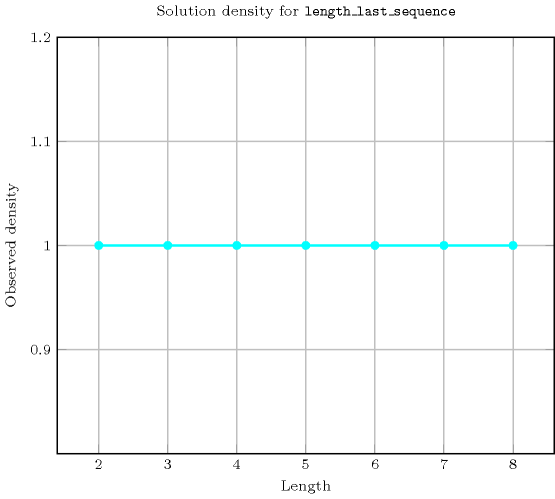Length ($n$)2345678
Total9646257776117649209715243046721
 Parameter value

16485006480100842183500838263752
23121001080144062293764251528
3-420180205828672472392
4--530294358452488
5---6424485832
6----756648
7-----872
8------9

Solution count for $\mathrm{𝚕𝚎𝚗𝚐𝚝𝚑}_\mathrm{𝚕𝚊𝚜𝚝}_\mathrm{𝚜𝚎𝚚𝚞𝚎𝚗𝚌𝚎}$: domains $0..n$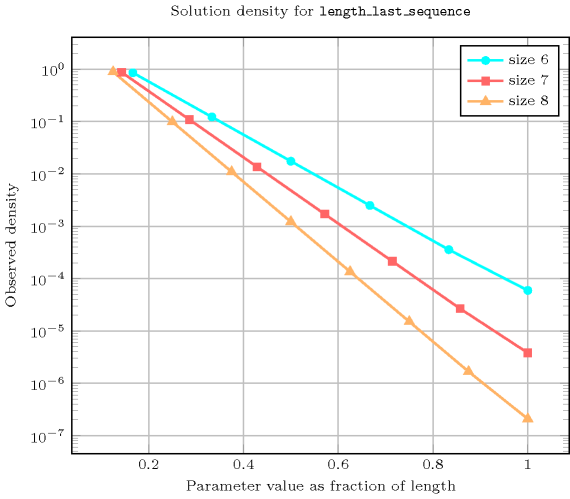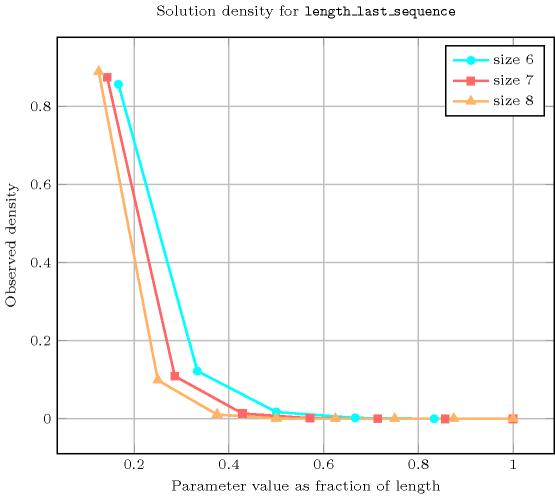Figure 5.216.1 depicts the automaton associated with the $\mathrm{𝚕𝚎𝚗𝚐𝚝𝚑}_\mathrm{𝚕𝚊𝚜𝚝}_\mathrm{𝚜𝚎𝚚𝚞𝚎𝚗𝚌𝚎}$ constraint. To each pair of consecutive variables $\left({\mathrm{𝚅𝙰𝚁}}_{i},{\mathrm{𝚅𝙰𝚁}}_{i+1}\right)$ of the collection $\mathrm{𝚅𝙰𝚁𝙸𝙰𝙱𝙻𝙴𝚂}$ corresponds a signature variable ${S}_{i}$. The following signature constraint links ${\mathrm{𝚅𝙰𝚁}}_{i}$, ${\mathrm{𝚅𝙰𝚁}}_{i+1}$ and ${S}_{i}$: ${\mathrm{𝚅𝙰𝚁}}_{i}={\mathrm{𝚅𝙰𝚁}}_{i+1}⇔{S}_{i}$.
##### Figure 5.216.1. Automaton of the $\mathrm{𝚕𝚎𝚗𝚐𝚝𝚑}_\mathrm{𝚕𝚊𝚜𝚝}_\mathrm{𝚜𝚎𝚚𝚞𝚎𝚗𝚌𝚎}$ constraint when $|\mathrm{𝚅𝙰𝚁𝙸𝙰𝙱𝙻𝙴𝚂}|\ge 2$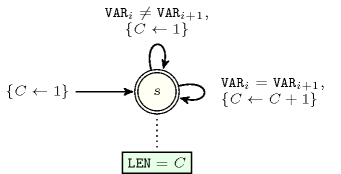##### Figure 5.216.2. Hypergraph of the reformulation corresponding to the automaton of the $\mathrm{𝚕𝚎𝚗𝚐𝚝𝚑}_\mathrm{𝚕𝚊𝚜𝚝}_\mathrm{𝚜𝚎𝚚𝚞𝚎𝚗𝚌𝚎}$ constraint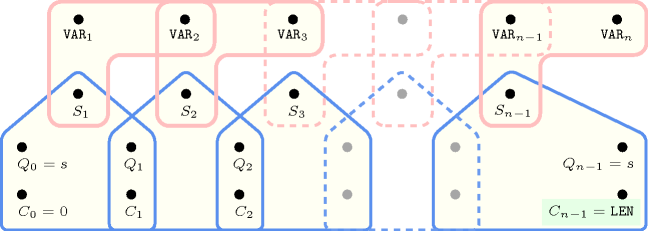##### Figure 5.216.3. Automaton of the reverse of the $\mathrm{𝚕𝚎𝚗𝚐𝚝𝚑}_\mathrm{𝚕𝚊𝚜𝚝}_\mathrm{𝚜𝚎𝚚𝚞𝚎𝚗𝚌𝚎}$ constraint (i.e., the $\mathrm{𝚕𝚎𝚗𝚐𝚝𝚑}_\mathrm{𝚏𝚒𝚛𝚜𝚝}_\mathrm{𝚜𝚎𝚚𝚞𝚎𝚗𝚌𝚎}$ constraint) when $|\mathrm{𝚅𝙰𝚁𝙸𝙰𝙱𝙻𝙴𝚂}|\ge 2$ and corresponding glue matrix between $\mathrm{𝚕𝚎𝚗𝚐𝚝𝚑}_\mathrm{𝚕𝚊𝚜𝚝}_\mathrm{𝚜𝚎𝚚𝚞𝚎𝚗𝚌𝚎}$ and its reverse $\mathrm{𝚕𝚎𝚗𝚐𝚝𝚑}_\mathrm{𝚏𝚒𝚛𝚜𝚝}_\mathrm{𝚜𝚎𝚚𝚞𝚎𝚗𝚌𝚎}$